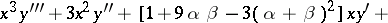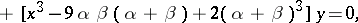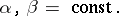# Bessel equation

(diff) ← Older revision | Latest revision (diff) | Newer revision → (diff)
Jump to: navigation, search

A second-order linear ordinary differential equation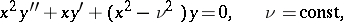(1)

or, in self-adjoint form: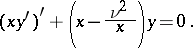The numberis called the order of the Bessel equation; in the general caseandassume complex values. The substitutionyields the reduced form of equation (1):(2)

A Bessel equation is a special case of a confluent hypergeometric equation; if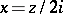is substituted into (2), equation (2) becomes a Whittaker equation. In equation (1) the point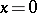is weakly singular, while the pointis strongly singular. For this reason a Bessel equation does not belong to the class of Fuchsian equations (cf. Fuchsian equation). F. Bessel  was the first to study equation (1) systematically, but such equations are encountered even earlier in the works of D. Bernoulli, L. Euler and J.L. Lagrange.

A Bessel equation results from separation of variables in many problems of mathematical physics , particularly in the case of boundary value problems of potential theory for a cylindrical domain.

The solutions of Bessel equations are called cylinder functions (or Bessel functions). These may be subdivided into the cylinder functions of the first kind (Bessel functions)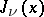, the cylinder functions of the second kind (Weber functions or Neumann functions, (cf. Weber function; Neumann function)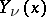and the cylinder functions of the third kind (Hankel functions)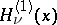,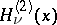. If the orderis fixed, all these functions are analytic functions of the complex argument; for all these functions, except for the functionsof integer order, the pointis a branch point. If the argumentis fixed, all these functions are single-valued entire functions of the complex order.

If the orderis not an integer, then the general solution of equation (1) may be written aswhere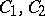are arbitrary constants. For a given order, any two of the functions,,,are linearly independent and may serve as a fundamental system of solutions of (1). For this reason, the general solution of equation (1) can be represented, in particular, in the following forms:The following equations are closely connected with equation (1): the equation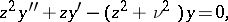which becomes (1) as a result of the substitution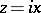, and with as a fundamental system of solutions the modified cylinder functions (Bessel functions of imaginary argument), and the equationwhich becomes equation (1) as a result of the substitutionand which has the Kelvin functions as its fundamental system of solutions. Many other second-order linear ordinary differential equations (e.g. the Airy equation) can also be transformed into equation (1) by a transformation of the unknown function and the independent variable. The solution of a series of linear equations of higher orders may be written in the form of Bessel functions .

The substitution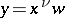transforms (1) into the Laplace equation: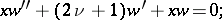which permits one to represent the solutions of (1) by contour integrals in the complex plane.

In applications it is often required to find the eigen values of the equation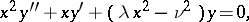(3)

whereis fixed whileis a parameter. Equation (3) on the segment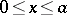with the boundary conditions: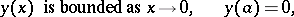is an example of a problem with a discrete spectrum (the eigen values are determined by the condition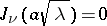in terms of the zeros of a Bessel function). Equation (3) with the boundary condition: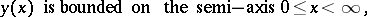represents a problem with a continuous spectrum (eigen values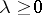).

The inhomogeneous Bessel equation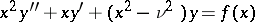(4)

has the particular solution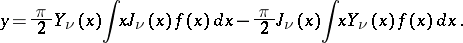Solutions of equation (4) have been studied in more detail for a right-hand side of special form. Thus, if, equation (4) is satisfied by a Lommel function; ifit is satisfied by a Struve function; if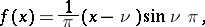it is satisfied by an Anger function; and ifit is satisfied by a Weber function.

There are linear equations of higher orders with solutions whose properties are analogous to those of Bessel functions. The general-th order equation of Bessel type has the form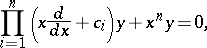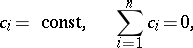and its solution depends onparameters. In particular, a third-order equation of Bessel type (which has a solution with two parameters,) may be represented in the form: